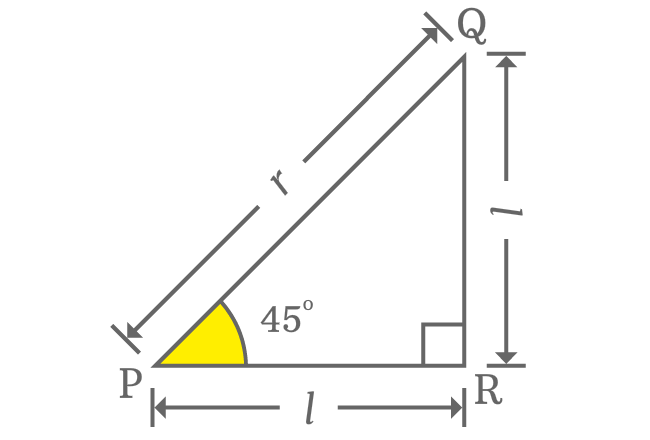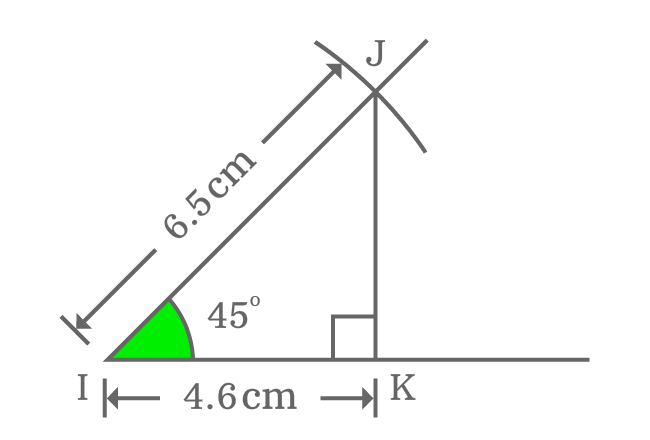# $\cos{(45^°)}$ Proof

The exact value of cos of $45$ degrees can be derived in mathematics in three methods, in which one method is trigonometric approach and other two are the slightly different geometrical approaches.

### Theoretical approach

Let’s remind the geometric property between adjacent and opposite sides when the angle of right triangle is $45$ degrees. According to this property, the lengths of adjacent and opposite sides are equal when angle of right angled triangle is equal to $45^°$. It helps us to evaluate the exact value of $\cos{(45^°)}$ in trigonometry theoretically.Take, the length of both adjacent and opposite side is denoted by $l$ and the length of hypotenuse is represented by $r$. Now, write Pythagorean Theorem in terms of the three sides of the right triangle.

${PQ}^2 \,=\, {PR}^2+{QR}^2$

$\implies r^2 = l^2 + l^2$

$\implies r^2 = 2l^2$

$\implies r = \sqrt{2}.l$

$\implies \dfrac{l}{r} = \dfrac{1}{\sqrt{2}}$

$l$ and $r$ are lengths of adjacent side (or opposite side) and hypotenuse respectively.

$\implies \dfrac{Length \, of \, Adjacent \, side}{Length \, of \, Hypotenuse} = \dfrac{1}{\sqrt{2}}$

When angle of right triangle is $45$ degrees, the ratio of lengths of adjacent side to hypotenuse is known as $\cos{\Big(\dfrac{\pi}{4}\Big)}$.

$\therefore \,\,\, \cos{(45^°)} = \dfrac{1}{\sqrt{2}}$

$\cos{(45^°)} = \dfrac{1}{\sqrt{2}} = 0.7071067812\ldots$

### Practical approach

You can also evaluate the value of cosine of angle $45$ degrees practically by constructing a right triangle with $45$ degrees angle by geometrical tools.1. Draw a straight horizontal line from Point $I$.
2. Coincide the point $I$ with centre of protractor and also coincide horizontal line with right side base line of the protractor. After that, mark a point at $45$ degrees.
3. Draw a straight line by ruler from point $I$ through $45$ degrees angle’s marked point.
4. Set compass to any length by ruler. In this case, compass is set to $6.5 \, cm$. Later, draw an arc on $45$ degrees angle line from point $I$ and it intersects the line at point $J$.
5. Lastly, a perpendicular line is drawn to horizontal line from point $J$ and it intersects the horizontal line at point $K$ perpendicularly. Thus, a right triangle, known as $\Delta JIK$ is formed geometrically.

It is time to calculate the value of $\cos{(50^g)}$ and it can be done by measuring the length of adjacent side of $\Delta JIK$ by a ruler.

$\cos{(45^°)} = \dfrac{Length \, of \, Adjacent \, side}{Length \, of \, Hypotenuse}$

$\implies \cos{(45^°)} \,=\, \dfrac{IK}{IJ}$The length of hypotenuse ($IJ$) is taken as $6.5 \, cm$ in this example. Take ruler and measure the length of adjacent side ($IK$). You will observe that the length of adjacent side is nearly $4.6 \, cm$. Now, find the ratio of lengths of adjacent side to hypotenuse to get the value of cos $45$ degrees in geometric approach on your own.

$\implies \cos{(45^°)} \,=\, \dfrac{IK}{IJ} = \dfrac{4.6}{6.5}$

$\,\,\, \therefore \,\,\,\,\,\, \cos{(45^°)} \,=\, 0.7076923077\ldots$

### Trigonometric approach

The exact value of $\cos{(50^g)}$ can also be evaluated in trigonometry by the cos squared formula. In this method, the value of sin $45$ degrees is substituted.

$\cos{(45^°)} \,=\, \sqrt{1-\sin^2{(45^°)}}$

$\implies \cos{(45^°)} \,=\, \sqrt{1-{\Bigg(\dfrac{1}{\sqrt{2}}\Bigg)}^2}$

$\implies \cos{(45^°)} \,=\, \sqrt{1-\dfrac{1}{2}}$

$\implies \cos{(45^°)} \,=\, \sqrt{\dfrac{1 \times 2 -1}{2}}$

$\implies \cos{(45^°)} \,=\, \sqrt{\dfrac{2-1}{2}}$

$\implies \cos{(45^°)} \,=\, \sqrt{\dfrac{1}{2}}$

$\,\,\, \therefore \,\,\,\,\,\, \cos{(45^°)} \,=\, \dfrac{1}{\sqrt{2}}$

#### Verdict

It is evaluated that the exact value of $\cos{\Big(\dfrac{\pi}{4}\Big)}$ is equal to $\dfrac{1}{\sqrt{2}}$ (or) $0.7071067812\ldots$ in both theoretical approaches of geometry and trigonometry but the same value is evaluated as $0.7076923077\ldots$ in practical geometric approach.

There is slight difference between values of both methods. It is also changed if you take different length of hypotenuse. The main reason for this is measuring error while measuring the length of adjacent side by ruler. It is very common in practical geometry but their approximate values are nearly same if you compare them.

In mathematics, the approximate value of $\cos{\Big(\dfrac{\pi}{4}\Big)}$ is considered as $0.7071$.

Latest Math Topics
Jun 26, 2023
Jun 23, 2023

###### Math Questions

The math problems with solutions to learn how to solve a problem.

Learn solutions

Practice now

###### Math Videos

The math videos tutorials with visual graphics to learn every concept.

Watch now

###### Subscribe us

Get the latest math updates from the Math Doubts by subscribing us.